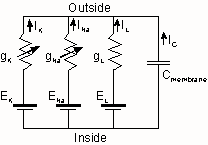## Hodgkin-Huxley model of squid axon

This program runs the Hodgkin-Huxley model of the squid giant axon in "current clamp" mode. Use it to see the voltage responses of the axon membrane to electrical currents.

#### Examples:

These examples show some characteristics of the model. Start with the default parameters and go through the steps in order. Click here get the default values back.

1. There is a hyperpolarization following an action potential.
2. Firing is all-or-none. This stimulus doesn't fire it.
3. But a slightly larger one does.
4. It can fire repetitively.
5. There is a refractory period after each action potential.
6. It fires anode break spikes.
7. Reset parameters to default.

In the late 1940's and early 1950's, Alan Hodgkin and Andrew Huxley used the newly-invented voltage clamp to investigate the ionic mechanism of the action potential. They found that the depolarizing phase of the action potential, or upstroke, is due to activation of sodium channels in the axonal membrane. Inward sodium ion flux tends to depolarize the axon.  The repolarizing phase, or downstroke, is due both to inactivation of sodium channels and activation of potassium channels. Reduced inward sodium flux coupled with outward flux of potassium ions causes repolarization.
They found that both sodium and potassium channels are activated, or gated, by voltage. Both are activated by depolarization of the axonal membrane. They were unable to study ion channel function at the single channel level, so they constructed a mathematical model of the ionic currents based on total ionic currents. The model can be described like an electric circuit with variable resistors representing the voltage-sensitive sodium and potassium conductances, a fixed capacitor representing the cell membrane, a fixed resistor representing a leakage conductance, and batteries representing the ionic potentials.In their model, sodium and potassium channel opening is determined by gates in the channel. The complexity of the model as a whole is due to the complexity of  the gating process, which determines the way in which the variable resistors in the circuit model operate. Sodium channels have two gates: one for activation and one for inactivation. Activation is proportional to the third power of a gating factor m, and inactivation is proportional to a gating factor h. Total sodium current is proportional to m3h, the fraction of the channels active. Potassium channels are hypothesized to have only one gate, and total potassium current is proportional to n4. These gating factors operate as a function of voltage and time.
Their voltage-clamp analysis satisfactorily predicted many of the features of the action potential in axons, including: the form, duration and amplitude of the spike; refractory period; subthreshold phenomena; and anode-break excitation.

### Parameters:

This program will allow you to control all of the parameters in the Hodgkin-Huxley model of the squid axon.

1. Integration parameters
1. step size
2. number of steps (number of data points)
3. step size for integration (Runge-Kutta method)
2. Stimulus current parameters
1. stimulus current amplitude
2. time at beginning of stimulus
3. stimulus duration
4. repetitive stimulation
5. stimulus rate
3. Axon parameters
1. initial membrane potential
2. resting membrane potential
3. specific membrane capacitance
4. Q10 for rate constants
5. temperature
6. maximal sodium conductance
7. sodium ion reversal potential
8. maximal potassium conductance
9. potassium ion reversal potential
10. leak conductance
11. leak current reversal potential
12. resting value of variable n
13. resting value of variable m
14. resting value of variable h
15. and the value of their rate equation constants

Back to neural models page